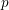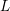Hostname: page-component-546b4f848f-zwmfq Total loading time: 0 Render date: 2023-06-04T16:04:54.830Z Has data issue: false Feature Flags: { "useRatesEcommerce": true } hasContentIssue false

# The$p$-adic$L$-functions of evil Eisenstein series

Published online by Cambridge University Press:  13 January 2015

## Abstract

HTML view is not available for this content. However, as you have access to this content, a full PDF is available via the ‘Save PDF’ action button.

We compute the$p$-adic$L$-functions of evil Eisenstein series, showing that they factor as products of two Kubota–Leopoldt$p$-adic$L$-functions times a logarithmic term. This proves in particular a conjecture of Glenn Stevens.

## MSC classification

Type
Research Article
Information
Compositio Mathematica , June 2015 , pp. 999 - 1040

## References

Ash, A. and Stevens, G., Modular forms in characteristic and special values of their L-functions, Duke Math. J. 53 (1986), 849868.CrossRefGoogle Scholar
Atkin, A. O. L. and Lehner, J., Hecke Operators on Γ0(m), Math. Ann. 185 (1970), 134160.CrossRefGoogle Scholar
Bellaïche, J.,$p$-adic$L$-functions of critical CM modular forms, available athttp://people.brandeis.edu/∼jbellaic/preprint/preprint.html.Google Scholar
Bellaïche, J., Introduction to the conjecture of Bloch and Kato, notes of lectures given at the CMI summer school in Hawaii, 2009, available athttp://people.brandeis.edu/∼jbellaic/preprint/preprint.html, to appear in the publications of the CMI.Google Scholar
Bellaïche, J., The eigencurve and$p$-adic$L$-functions, notes of a course given at BrandeisUniversity, Fall 2010, available at http://people.brandeis.edu/∼jbellaic/preprint/preprint.html .Google Scholar
Bellaïche, J. and Chenevier, G., Lissité de la courbe de Hecke de GL(2) aux points Eisenstein critiques, J. Inst. Math. Jussieu 5 (2006), 333349.CrossRefGoogle Scholar
Buzzard, K., Eigenvarieties, in L-functions and Galois representations, London Mathematical Society Lecture Note Series, vol. 320 (Cambridge University Press, Cambridge, 2007), 59120.CrossRefGoogle Scholar
Chenevier, G., Une correspondance de Jacquet–Langlands p-adique, Duke Math. J. 126 (2005), 161194.CrossRefGoogle Scholar
Coleman, R., Classical and overconvergent modular forms, Invent. Math. 124 (1996), 215241.CrossRefGoogle Scholar
Colmez, P., Fonctions Lp-adiques, in Séminaire Bourbaki, Vol. 1998/99, Astérisque, vol. 266 (Société Mathématique de France, 2000), 2158; Exp. No. 851, 3.Google Scholar
Darmon, H. and Dasgupta, S., Elliptic units for real quadratic fields, Ann. of Math. (2) 163 (2006), 301345.CrossRefGoogle Scholar
Dasgupta, S., Gross–Stark units, Stark–Heegner points, and class fields of real quadratic fields, PhD thesis, University of California, Berkeley (2004).Google Scholar
Diamond, F. and Shurman, J., A first course in modular forms, Graduate Texts in Mathematics, vol. 228 (Springer, New York, 2005).Google Scholar
Emerton, M., Pollack, R. and Weston, T., Variation of Iwasawa invariants in Hida families, Invent. Math. 163 (2006), 523580.CrossRefGoogle Scholar
Greenberg, R. and Stevens, G., p-adic L-functions and p-adic periods of modular forms, Invent. Math. 111 (1993), 407447.CrossRefGoogle Scholar
Hida, H., Elementary theory of L-functions and Eisenstein series, London Mathematical Society Student Texts, vol. 26 (Cambridge University Press, Cambridge, 1993).CrossRefGoogle Scholar
Jannsen, U., On the l-adic cohomology of varieties over number fields and its Galois cohomology, in Galois groups over ℚ (Berkeley, CA, 1987), Mathematical Sciences Research Institute Publications, vol. 16 (Springer, New York, 1989), 315360.CrossRefGoogle Scholar
Kostadinov, K., Constructing an explicit modular symbol, PhD thesis, Boston University (2010).Google Scholar
Mazur, B., Tate, J. and Teitelbaum, J., On p-adic analogues of the conjectures of Birch and Swinnerton-Dyer, Invent. Math. 84 (1986), 148.CrossRefGoogle Scholar
Miyake, T., Modular forms (Springer, Berlin, 1976).Google Scholar
Pollack, R. and Stevens, G., Critical slope p-adic L-functions, J. Lond. Math. Soc. (2) 87 (2013), 428452.CrossRefGoogle Scholar
Stein, W., Modular forms, inA computational approach, Graduate Studies in Mathematics, vol. 79 (American Mathematical Society, Providence, RI, 2007), available athttp://modular.math.washington.edu/books/modform/modform/index.html.Google Scholar
Stevens, G., Rigid analytic modular symbols, Preprint, available athttp://math.bu.edu/people/ghs/research.d.Google Scholar